# An Implementation of the Marching Cubes Algorithm

By Ben Anderson

#### Abstract:

The Marching cubes algorithm can be described as follows:
Given an object, a test to determine whether an arbitrary point is within the object, and bounds within which the object exists:
Divide the space within the bounds into an arbitrary number of cubes.  Test the corners of every cube for whether they are inside the object.  For every cube where some corners are inside and some corners are outside the object, the surface must pass through that cube, intersecting the edges of the cube in between corners of opposite classification.  Draw a surface within each cube connecting these intersections.  You have your object.

#### Full Text:

Once we have masked images of every slice of the object we are to reconstruct (ie, a black and white image where black is inside and white is outside the object), we can go about reconstructing the original surface.  One way of doing this is to use the Marching Cubes algorithm.

### In 2D:

To understand how the Marching Cubes algorithm works, let's take a 2D case and what might be called the "Marching Squares" algorithm.  Here's our object:Now, let's divide it into squares: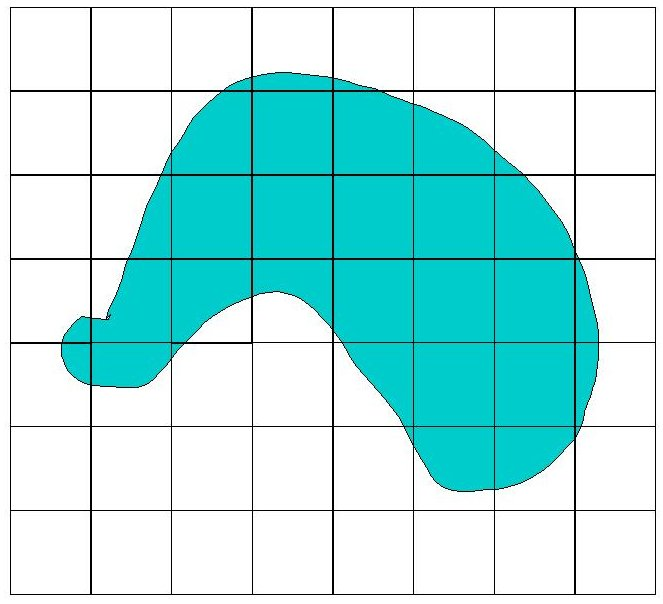We can tell by looking at it which vertices are in, and which are outside of the object, so let's label them, red for inside, blue for out:Now we know that somewhere on each edge between an inside corner and an outside one the original surface must intersect our squares (purple dots):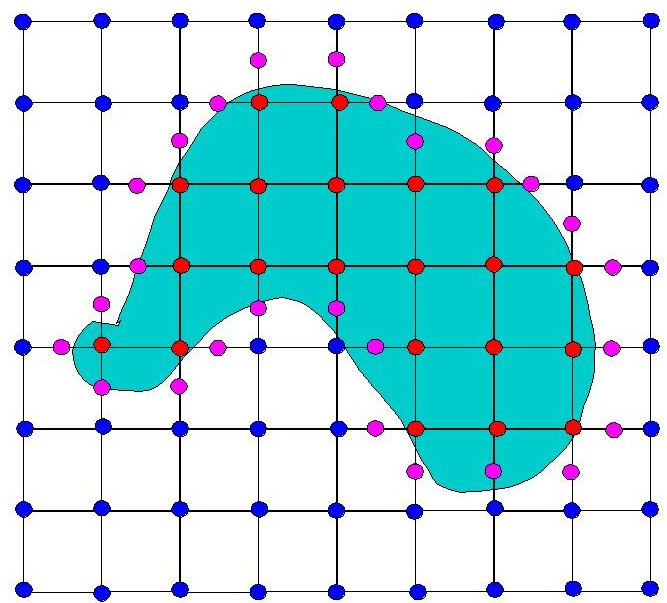Within each square, let's connect the purple dots.  Now we have an approximation of the original surface (the purple lines):## In 3D:

Implementing the algorithm in 3D works much the same as it did in 2D.  For slice data like the Visible Human Male dataset, you stack the slices in 3D, knowing each slice is 1mm or 3 pixels appart.  In order to be able to test the vertices of each cube, you must choose your cube size to align with the slices, either using 1mm cubes (or rectangles 1mm high and 1/3rd of a mm thick since pixels are only 1/3rd of a mm wide), or some multiple of 1mm cubes (ie 2mm or 3 mm) so that each horizontal side of your cubes falls on the plane of a slice.  You then can test each vertex by going to the masked slices corresponding to each cube's top and bottom z values.  You now have a bunch of cubes with labeled corners.  For each cube, you know the surface intersects the cube along the edges in between corners of opposing classifications.  Each cube should look something like this: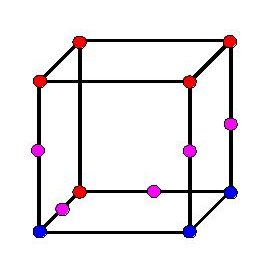In 2D to approximate the surface we simply had to draw lines between each purple dot.  In 3D this would give us a strange line drawing like this:Which doesn't look very much like a piece of a circle.  Instead, what we want to do is triangulate the cube where filled triangles will represent the surface passing through the cube.  Something like
this: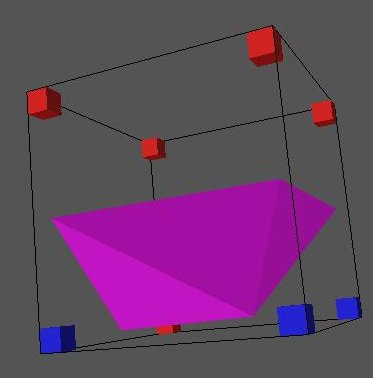However, you'll note that this isn't as easy as drawing a line connecting all the purple dots in a square.  For one thing, a triangle connects 3 dots, and for another, it's hard to tell which three dots to connect in which triangle.  If you just randomly connected dots in a triangle, you might get something like: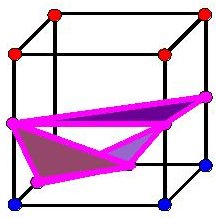Which really doesn't look any good.  The other issue is that since you're going to be doing this to a lot of cubes, you probably want whatever solution you find to be fast.  And what is really fast?  A lookup table.  Consider a cube.  Each corner can be either inside or outside the object.  There are eight corners.  That means you're working with 28, or 256 different possibilities for what a cube might look like, which is definitely feasible.  Triangulating each of those possibilities might be a pain, but luckily, given identical triangulations for cubes whose vertex classification is opposite of eachother, rotations and mirroring, there are in fact only 14 unique triangulations: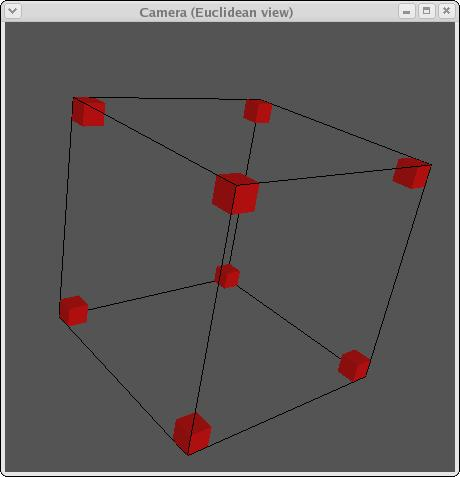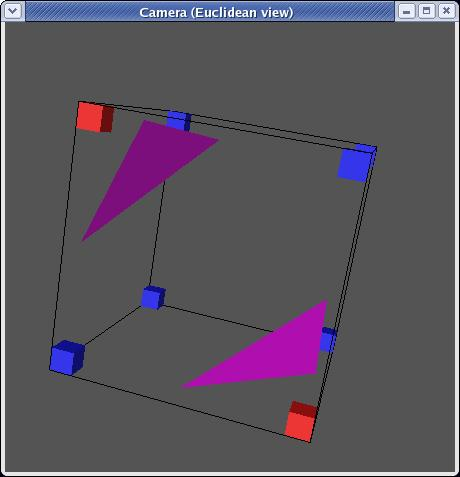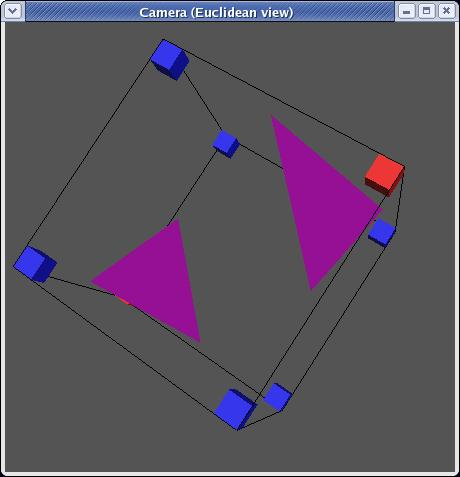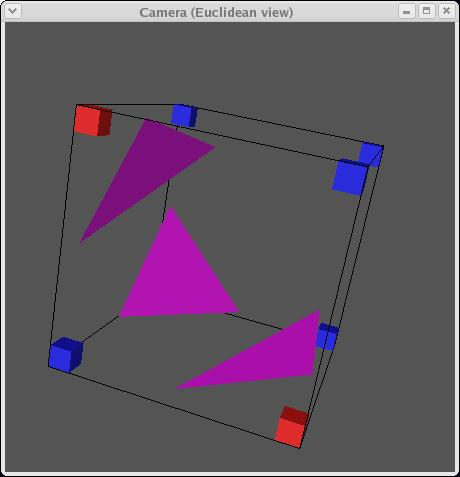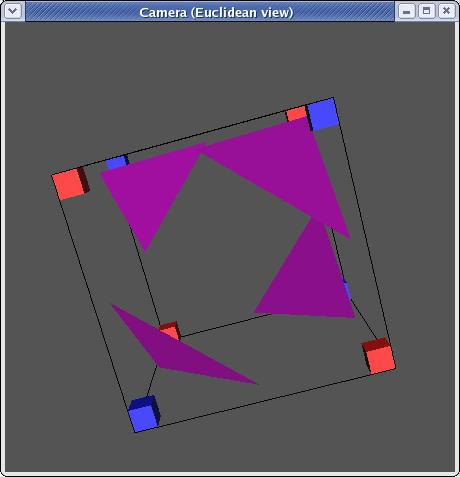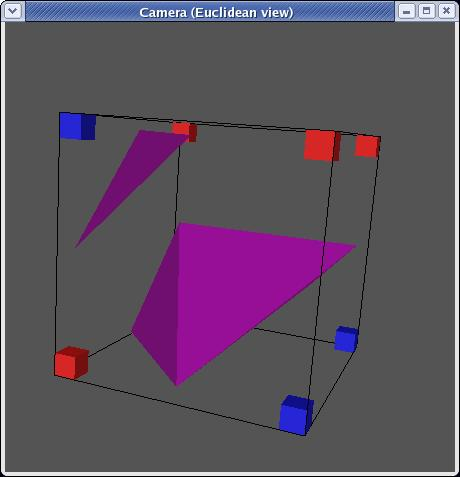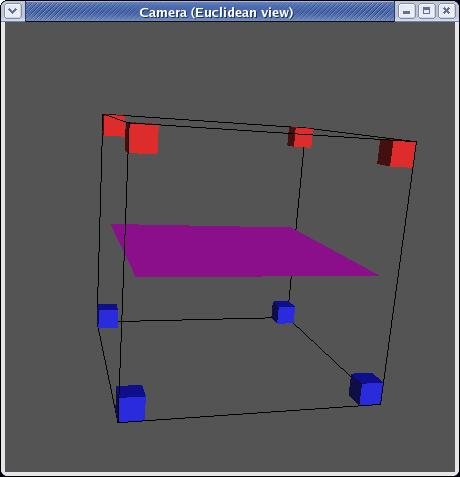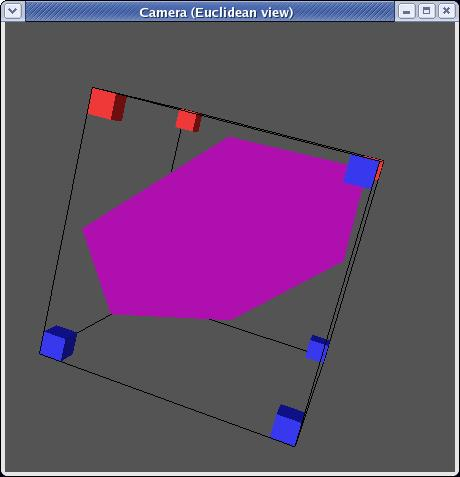Each triangulation contains zero to four triangles and can be stored as a list of triangles where each triangle is a list of 3 numbers which are indexes to the edges on which each triangles vertices lie. for an example, here's some ASCII art taken from the code labeling each edge and each corner with an index:

//                 v7_______e6_____________v6
//                  /|                    /|
//                 / |                   / |
//              e7/  |                e5/  |
//               /___|______e4_________/   |
//            v4|    |                 |v5 |e10
//              |    |                 |   |
//              |    |e11              |e9 |
//            e8|    |                 |   |
//              |    |_________________|___|
//              |   / v3      e2       |   /v2
//              |  /                   |  /
//              | /e3                  | /e1
//              |/_____________________|/
//              v0         e0          v1

As you can see, the triangle in the second example might be defined as [e3,e2,e11].  A cube can be looked up in the table by taking the classification of each vertex and converting it to 0's and 1's and then forming a binary number.  Again, using the same cube as an example, v3 is inside the shape and every other vertex is out.  This results in a binary number of 00010000 or 23 or 8, so the entry for 8 in the lookup table might be:

8: [e1,e2,e11]

In the actual code, it doesn't look quite like that, but you get the idea.

And so, in order to compute the triangulation for the entire image, you do this for every cube, offset the triangulations to their appropriate locations in the space and voila!As you can tell, this is a blocky rendering of a foot.  You were probably hoping for somthing better.  Well, luckily, this is only rendered using 1/3rd of the slices, (fairly big cubes).  To overcome this, the first thing you might think of is to make the cubes smaller resulting in a more accurate rendering of a foot:Well, that's better, but as you can tell, it's still fairly blocky.  Well, we can do better.  To understand how, let's go back to 2D.

Here's our approximation in 2D from earlier:Now, without even using smaller cubes, we can come up with a much better approximation of this surface, and one that's much smoother (the surface right now is made up of facets meeting at 45° angles).  How can we do that?  Like this:The only difference here is that the purple dots have been moved.  Instead of placing the purple dots midway between the red and the blue ones, they have been moved to meet up with the actual intersection of the surface with the edges in question.  This results in a more accurate representation of the surface, as well as a smoother one using the same number of cubes as before.  Let's see what that looks like in 3D: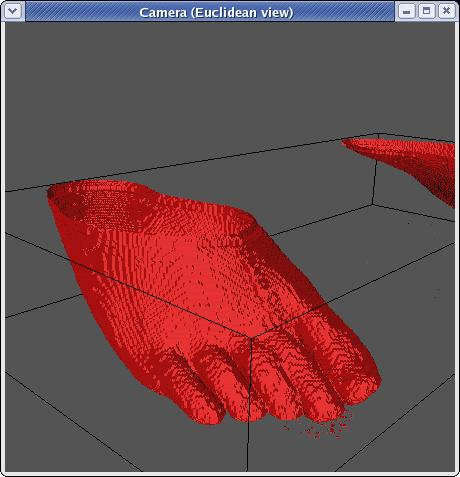Now, that is smoother, and you can almost make out the toenail ont he big toe, but if you're saying to yourself, "That's still pretty blocky," you'd be right.

The main problem is that while in 2D we can compute exact intersections for every edge of our shape, given only slice data, in 3D, we cannot do this.  Take this picture for example: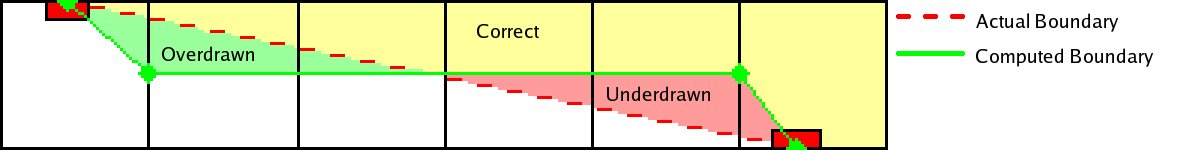This is a side view of a row of cubes.  The top line is a slice, as is the bottom.  The problem is that while we can compute exact intersections on a given slice, in between, we have no idea where the
object lies.  While the actual surface is represented by the dotted red line, in between layers, we have no information as to where that line intersects the cubes.  The only choice is to guess and place it midway between slices given that in any single cube, the only information you have between layers is that on top of the cube every point is inside and on the bottom, every point is out.  This results in a stairstepping effect particularly apparent when the surface becomes more horizontal (closer to parallel with the slices) and there is a greater horizontal distance (a larger number of cubes) between intersections with the cutting planes.   What we get is an effect like this:Luckily, there's still more we can do.

One step would be to take a trick from CS 317 (Graphics) and use Gouraud Shading to render the object.  For those of you who don't know what that is, you'll have to take Jack Goldfeather's course next year.  A basic explanation is that for each vertex, you can compute an average surface normal at that point by averaging the normals of all triangles sharing that vertex.  At each vertex then, you can use that normal to compute the lighting at that point.  Given the lighting at each vertex of a triangle, you can interpolate for every point between to determine how bright the surface is at that point.  The overall effect is to blur the appearance of edges without changing the actual geometry of the object.  Doing this we get:Which is smoother still, but still not quite what we'd like.  To do better we're going to have to work with a little post-processing.

## Coloring the Visible Human

So far all the images we've seen have been red.  As you know, feet are not red.  There are two basic options for coloring reconstructions.  One is to simply use a more appropriate color:As you can see, this is significantly more foot like.  The second option is to use the actual color of the images just inside the point of intersection:This also looks footlike.  The bronze color from the first image was actually taken from one of the rgb values in the back of the foot.  However, as you can see from the blue tint on the front of the foot, taking the actual rgb values has the unfortunate side effect of grabbing values that contain some of the blue from the ice.  This is partly because of the fact that the threshold for being outside the object is 60% blue (rather than 50%) and partly because the rgb values for vertices in between slices are arbitrarily taken from one of the slices and sometimes that slice at that xy value is blue.

## Analysis:

The marching cubes algorithm is very well suited to surface reconstruction.  Given a surface for which you can test arbitrary points for whether they fall inside or outside the object, it's only weakness is occasional extraneous triangles.  It is fast (linear increases in time as area increases), accurate and works with arbitrarily shaped objects.  With slice data it's only additional weakness is a stairstepping effect when the surface approaches parallel with the slices.  Both of these weaknesses can be overcome with post processing.

For much cooler pictures, check out our results.

References:
1. Lorensen, W. E. and Cline, H. E., "Marching Cubes: A High Resolution 3D Surface Construction Algorithm," Computer Graphics, vol. 21, no. 3, pp. 163-169, July 1987.
2. Lorensen, W. E., "Marching Through the Visible Man," IEEE Visualization, Proceedings of the 6th conference on Visualization '95, pp. 368-373. 1994.
3. Lorensen, W. E., Schroeder, W. J. and Zarge, Jonathan A., "Decimation of Triangle Meshes," Internationa Conference on Computer Graphics and Interactive Techniques, Proceedings of the 19th annual conference on Computer Graphics and Interactive Techniques, pp. 65-70. 1992.
4. Taubin, G., "Curve and Surface Smoothing without Shrinkage," IBM Research Report RC-19536, September 1994.